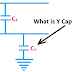Hey, in this article we are going to know what is Y Capacitor and what are the function and uses of Y Capacitor. There is a very important role of Y capacitors in power supply designing or power supply circuits. When you are going to learn about SMPS circuits, inverter circuits, rectifier circuits, or other high power supply circuits, you will hear the term 'Y Capacitor'. So, let's about the Y capacitor.

## What is Y Capacitor?

Y Capacitor is nothing but a bypass capacitor that is used to reduce Electromagnetic Magnetic Interference(EMI). The Y capacitor also can be called a filter capacitor. There are two types of filter or safety capacitors - X capacitor and Y capacitor. Generally, the Y capacitors are connected between line and earth terminals, also the neutral and earth terminals of a power supply circuit.

Y capacitors are mostly used in SMPS(Switched Mode Power Supply) Circuits. There are a lot of reasons for the production of electromagnetic interference such as the high-frequency switching mechanism of inverter circuits built with MOSFET or IGBT, Parasitic Capacitance between PCB tracks, parasitic capacitance between collector or drain and heat sink, rectifier circuit diodes, etc. Various types of inter-winding and Intra-winding capacitance of the SMPS transformer also produce electromagnetic interference. Y capacitor can reduce the electromagnetic interference produced by those sources.

## How Y Capacitor Works?

The working principle of a Y Capacitor is very simple. We know, the reactance of the capacitor decrease with the increase of frequency. So, when electromagnetic interference occurs, a very high frequency of unwanted signal or current is produced. So, these high-frequency noise signals bypass or grounded through the Y capacitor. Thus the electromagnetic interference is also eliminated.

Remember that one terminal of the Y Capacitor is always connected to the earth and the other terminal of the Y Capacitor is connected to Line and/or neutral. Y capacitors are very high-value capacitors. In normal conditions, they do not allow any through them, which means in normal conditions, Y capacitors provide complete isolation between line and earth, also neutral and earth. When the electromagnetic occurs, it provides a very low impedance path between the line and earth or neutral and earth, so the noise current is easily grounded.

## Application and Uses of Y Capacitor

The main function of a Y Capacitor is to eliminate electromagnetic interference by grounding the unwanted high frequency noisy signals. So, the Y capacitors are mostly used in SMPS circuits, sine wave inverter circuits, chopper circuits, etc.
The Y capacitor bypasses the leakage and noisy current and prevents it from going to LISN Resistor. Y capacitor also reduces the common-mode(CM) noise signals. Y capacitors are mostly used in DC circuit than AC circuits.

## Y Capacitor Value and Rating

According to the type of bridge insulation, Y capacitor is classified into different types and they are rated to use for different voltage levels. Such as Y1 capacitors are rated upto 500V AC, Y2 capacitors are rated from 150V AC to 300V AC, Y3 capacitors are rated to 250V AC, Y4 capacitors are rated up to 150V AC. According to the system voltage level, a proper type of Y capacitor is used. Selection of proper rating of Y capacitor is very important otherwise it can produce leakage current itself.

What is Y Capacitor? Applications, Uses, ValuesReviewed by Author on August 06, 2021 Rating: 5